Next: SLA_RVEROT - RV Corrn to Earth Centre
Up: SUBPROGRAM SPECIFICATIONS
Previous: SLA_REFV - Apply Refraction to Vector

## SLA_REFZ - Apply Refraction to ZD

ACTION:
Adjust an unrefracted zenith distance to include the effect of atmospheric refraction, using the simple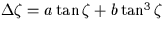model.

CALL:
CALL sla_REFZ (ZU, REFA, REFB, ZR)

GIVEN:

 D ZU unrefracted zenith distance of the source (radians) REFA D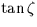coefficient (radians) REFB D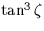coefficient (radians)

RETURNED:

 D ZR refracted zenith distance (radians)

NOTES:
1.
This routine applies the adjustment for refraction in the opposite sense to the usual one - it takes an unrefracted (in vacuo) position and produces an observed (refracted) position, whereas themodel strictly applies to the case where an observed position is to have the refraction removed. The unrefracted to refracted case is harder, and requires an inverted form of the text-book refraction models; the formula used here is based on the Newton-Raphson method. For the utmost numerical consistency with the refracted to unrefracted model, two iterations are carried out, achieving agreement at the 10-11 arcsecond level for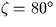. The inherent accuracy of the model is, of course, far worse than this - see the documentation for sla_REFCO for more information.
2.
At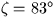, the rapidly-worseningmodel is abandoned and an empirical formula takes over: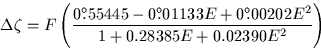where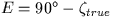and F is a factor chosen to meet theformula at. Over a wide range of observer heights and corresponding temperatures and pressures, the following levels of accuracy are achieved, relative to numerical integration through a model atmosphere:

 error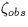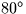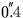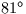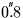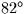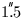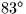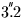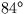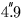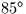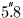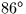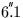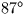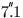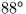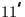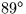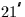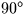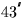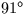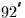< high-altitude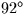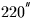< sites only
3.
See also the routine sla_REFV, which performs the adjustment in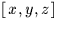, and with the emphasis on speed rather than numerical accuracy.Next: SLA_RVEROT - RV Corrn to Earth Centre
Up: SUBPROGRAM SPECIFICATIONS
Previous: SLA_REFV - Apply Refraction to Vector

SLALIB --- Positional Astronomy Library